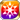# The ConquerorA book cannot be judged by its cover. In the same way, Orion is more than he seems. Rough he may be, on the outside, but this only serves to disguise his calculating mind. A shame that more do not realize this fact, for it is to their detriment. He cannot be underestimated, for he will take all in this world as his own. "Kuza, you may come with me if you wish to see something you have never seen before." There will never be anyone quite like this man, nor any who can understand battle tactics as he does. Yet, perhaps with my knowledge, I could be the one to make his ambitions a reality. (From the Preface of "A Chronicle of a Life", by Kuza)

## Stats

#### Simple stats

TypeLvl 1Lvl 30Lvl 40
AGI+2+5+7

LevelAGI
1+2
2+2
3+2
4+2
5+2
6+2
7+2
8+2
9+3
10+3
LevelAGI
11+3
12+3
13+3
14+3
15+3
16+3
17+4
18+4
19+4
20+4
LevelAGI
21+4
22+4
23+4
24+4
25+5
26+5
27+5
28+5
29+5
30+5
LevelAGI
31+5
32+5
33+6
34+6
35+6
36+6
37+6
38+6
39+6
40+7

## Group skills

### Greed Dike's Defense

#### Simple stats

TypeLvl 1Lvl 30Lvl 40
PDEF+13+40+50

LevelPDEF
1+13
2+13
3+14
4+15
5+16
6+17
7+18
8+19
9+20
10+21
LevelPDEF
11+22
12+23
13+24
14+25
15+26
16+27
17+28
18+29
19+30
20+31
LevelPDEF
21+31
22+32
23+33
24+34
25+35
26+36
27+37
28+38
29+39
30+40
LevelPDEF
31+41
32+42
33+43
34+44
35+45
36+46
37+47
38+48
39+49
40+50

#### Limit Break

TypeLB1LB2LB3LB4LB5
PDEF+6+12+18+24+30

### Greed Dike's Defense

#### Simple stats

TypeLvl 1Lvl 30Lvl 40
PDEF+13+40+50

LevelPDEF
1+13
2+13
3+14
4+15
5+16
6+17
7+18
8+19
9+20
10+21
LevelPDEF
11+22
12+23
13+24
14+25
15+26
16+27
17+28
18+29
19+30
20+31
LevelPDEF
21+31
22+32
23+33
24+34
25+35
26+36
27+37
28+38
29+39
30+40
LevelPDEF
31+41
32+42
33+43
34+44
35+45
36+46
37+47
38+48
39+49
40+50

#### Limit Break

TypeLB1LB2LB3LB4LB5
PDEF+6+12+18+24+30

### Alliance Without a Future

#### Simple stats

TypeLvl 1Lvl 30Lvl 40
Jewels Obtained+4+12+15
Hit Rate+4+12+15
HP+125+403+500

#### Detailed stats

LevelJewels ObtainedHit RateHP
1+4+4+125
2+4+4+134
3+4+4+144
4+4+4+153
5+5+5+163
6+5+5+173
7+5+5+182
8+5+5+192
9+6+6+201
10+6+6+211
LevelJewels ObtainedHit RateHP
11+6+6+221
12+7+7+230
13+7+7+240
14+7+7+250
15+7+7+259
16+8+8+269
17+8+8+278
18+8+8+288
19+9+9+298
20+9+9+307
LevelJewels ObtainedHit RateHP
21+9+9+317
22+9+9+326
23+10+10+336
24+10+10+346
25+10+10+355
26+11+11+365
27+11+11+375
28+11+11+384
29+11+11+394
30+12+12+403
LevelJewels ObtainedHit RateHP
31+12+12+413
32+12+12+423
33+13+13+432
34+13+13+442
35+13+13+451
36+13+13+461
37+14+14+471
38+14+14+480
39+14+14+490
40+15+15+500

#### Limit Break

TypeLB1LB2LB3LB4LB5
Jewels Obtained+2+3+5+7+9
Hit Rate+2+3+5+7+9
HP+75+131+187+243+300

TypeLB5
Area ATK Res+8

### All in My Grasp

#### Simple stats

TypeLvl 1Lvl 30Lvl 40
PATK+15+48+59
MATK+15+48+59
Hit Rate+3+8+10

#### Detailed stats

LevelPATKMATKHit Rate
1+15+15+3
2+16+16+3
3+17+17+3
4+18+18+3
5+19+19+3
6+20+20+3
7+21+21+4
8+23+23+4
9+24+24+4
10+25+25+4
LevelPATKMATKHit Rate
11+26+26+4
12+27+27+4
13+28+28+5
14+30+30+5
15+31+31+5
16+32+32+5
17+33+33+5
18+34+34+6
19+35+35+6
20+36+36+6
LevelPATKMATKHit Rate
21+38+38+6
22+39+39+6
23+40+40+6
24+41+41+7
25+42+42+7
26+43+43+7
27+45+45+7
28+46+46+7
29+47+47+8
30+48+48+8
LevelPATKMATKHit Rate
31+49+49+8
32+50+50+8
33+51+51+8
34+53+53+8
35+54+54+9
36+55+55+9
37+56+56+9
38+57+57+9
39+58+58+9
40+59+59+10

#### Limit Break

TypeLB1LB2LB3LB4LB5
PATK+7+14+21+28+36
MATK+7+14+21+28+36
Hit Rate+1+2+3+4+6

TypeLB5
Thunder+10

### Wings of Supremacy

#### Simple stats

TypeLvl 1Lvl 30Lvl 40
Area ATK+3+6+8
Hit Rate+5+12+15

#### Detailed stats

LevelArea ATKHit Rate
1+3+5
2+3+5
3+3+5
4+3+5
5+3+6
6+3+6
7+3+6
8+3+6
9+4+7
10+4+7
LevelArea ATKHit Rate
11+4+7
12+4+7
13+4+8
14+4+8
15+4+8
16+4+8
17+5+9
18+5+9
19+5+9
20+5+9
LevelArea ATKHit Rate
21+5+10
22+5+10
23+5+10
24+5+10
25+6+11
26+6+11
27+6+11
28+6+11
29+6+12
30+6+12
LevelArea ATKHit Rate
31+6+12
32+6+12
33+7+13
34+7+13
35+7+13
36+7+13
37+7+14
38+7+14
39+7+14
40+8+15

#### Limit Break

TypeLB1LB2LB3LB4LB5
Area ATK+1+2+3+4+5
Hit Rate+2+3+5+7+9

#### Max Limit Break

TypeLB5
Strong vs Light+20

## Vision abilities

### Limit Reached Bonus

#### Ability base

• Slot:Support

Revealed after Limit Reached. Raises AGI after appearing on map.

#### Ability max

• Slot:Action

Raises AGI after appearing on map.